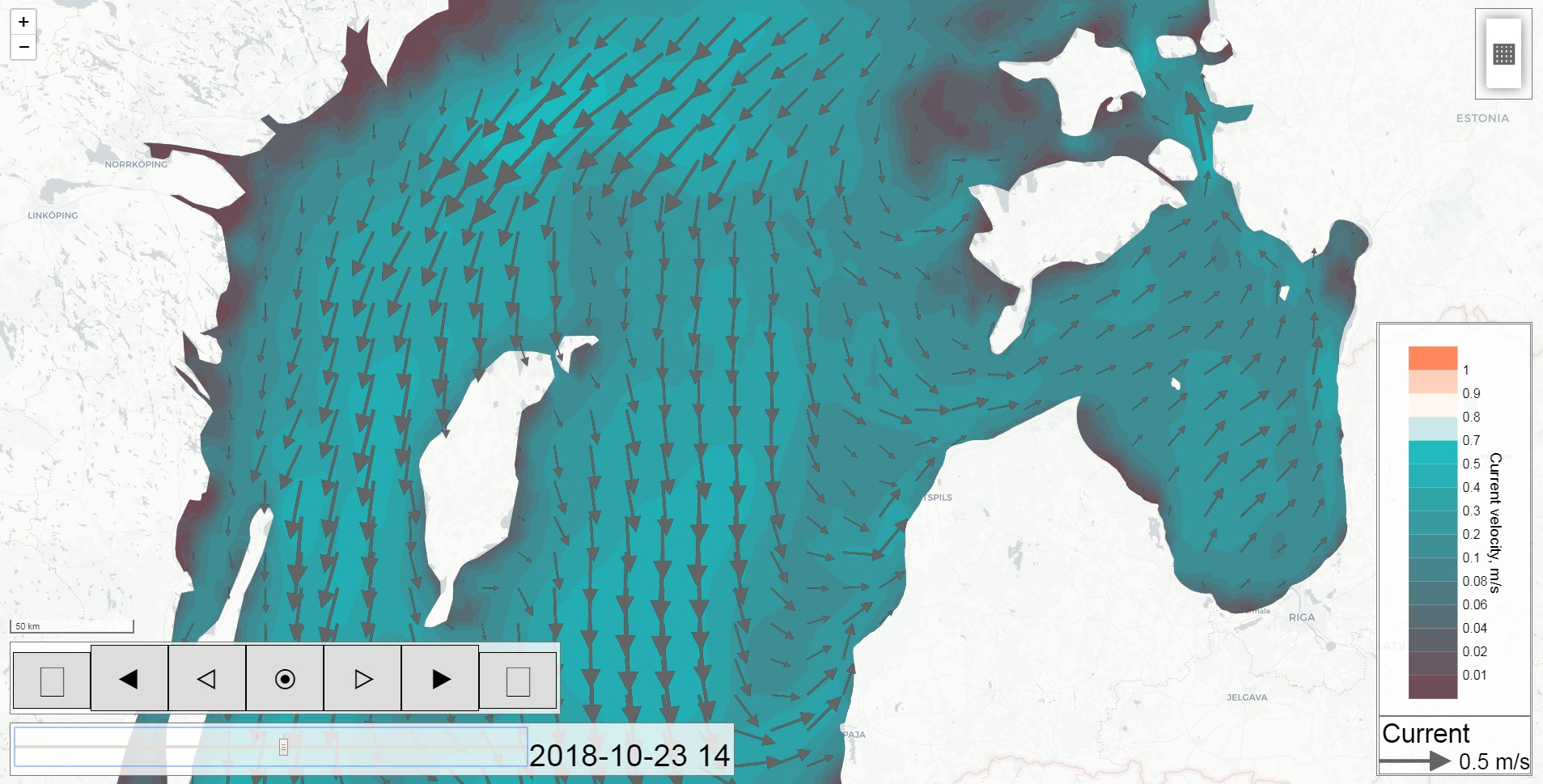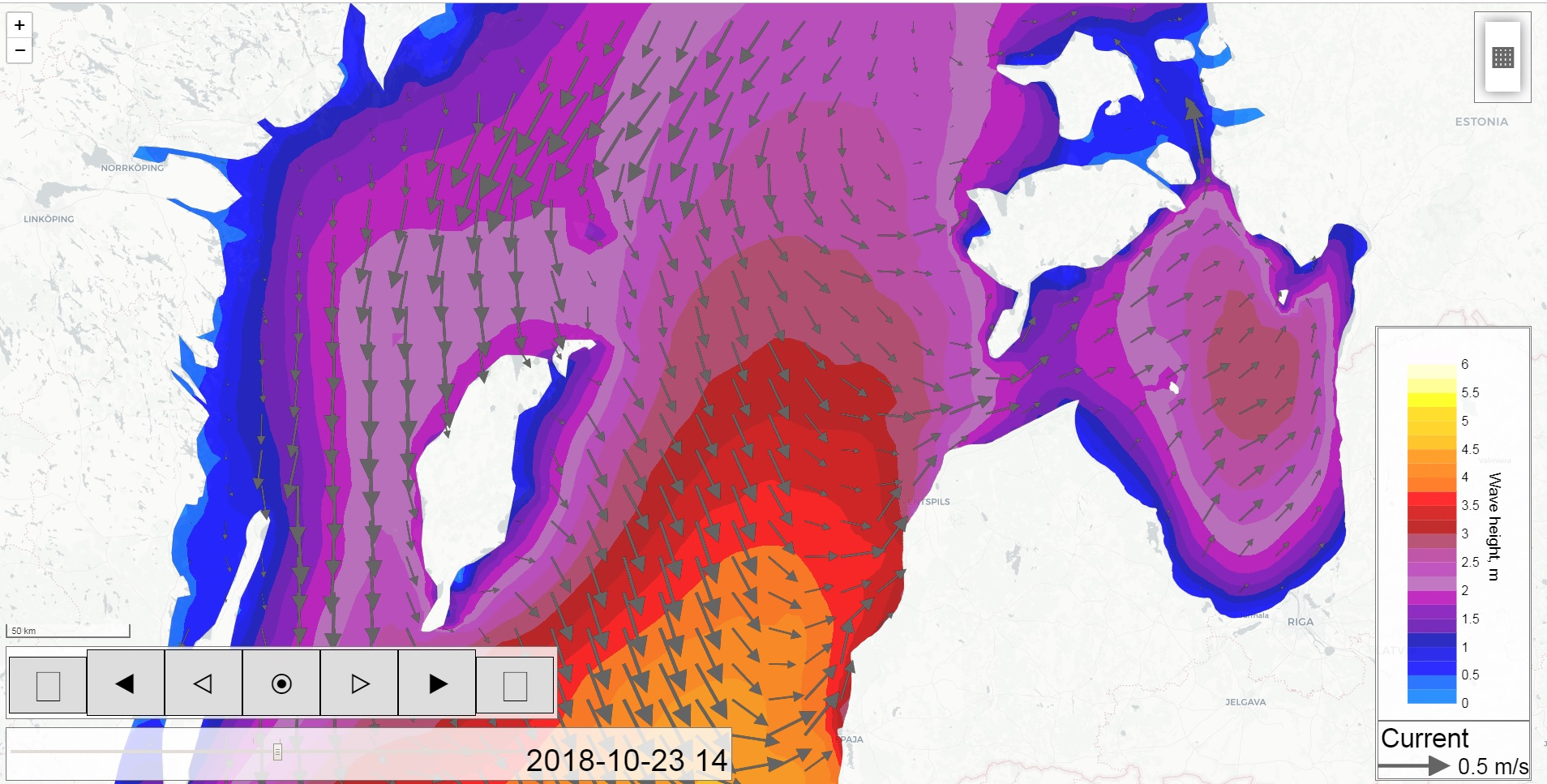"Present the present" current velocity plot example"Present the present" water level plot example"Present the present" wave height plot example"Present the present" water temperature plot example"Present the present" salinity plot example"Present the present" wind speed plot example